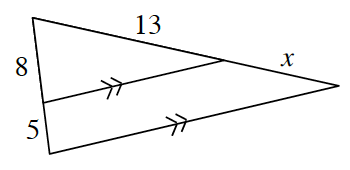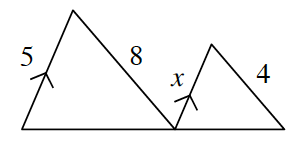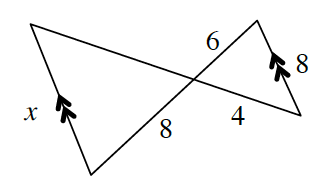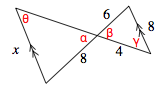### Home > CCG > Chapter 6 > Lesson 6.2.5 > Problem6-96

6-96.

For each part below, decide if the triangles are similar. If they are similar, use their similarity to solve for $x$. If they are not similar, explain why not.

1.The triangles share a common angle and parallel lines have congruent corresponding angles, so the triangles are similar by $\text{AA}\sim$.

$\frac{8}{13} = \frac{13}{13 + x}$

$\begin{array}{l} 8(13+x)=13·13\\ \;104+8x=169\\ \qquad \quad 8x=65 \end{array}$

$x=\frac{65}{8}$

1.Is there enough information to prove that the triangles are similar?

1.Vertical angles are congruent, so $α=β$.
Parallel lines have congruent alternate interior angles, so $θ=\text{Y}$.
Triangles are similar by $\text{AA}\sim$.

$\begin{array}{l} \frac{6}{8} = \frac{8}{x} \\ 6x = 8(8) \\ 6x = 64 \\ \; \;x \approx 10.67 \end{array}$